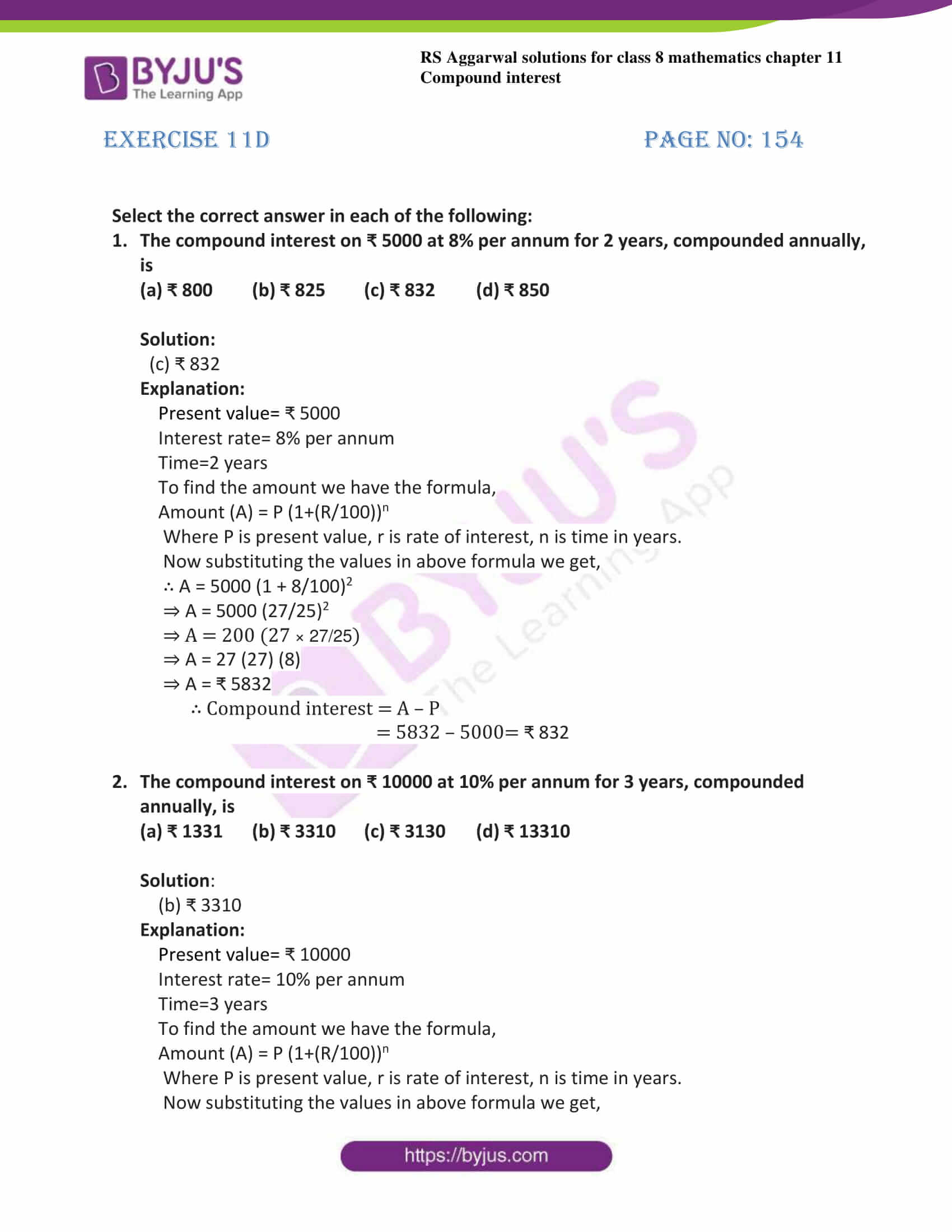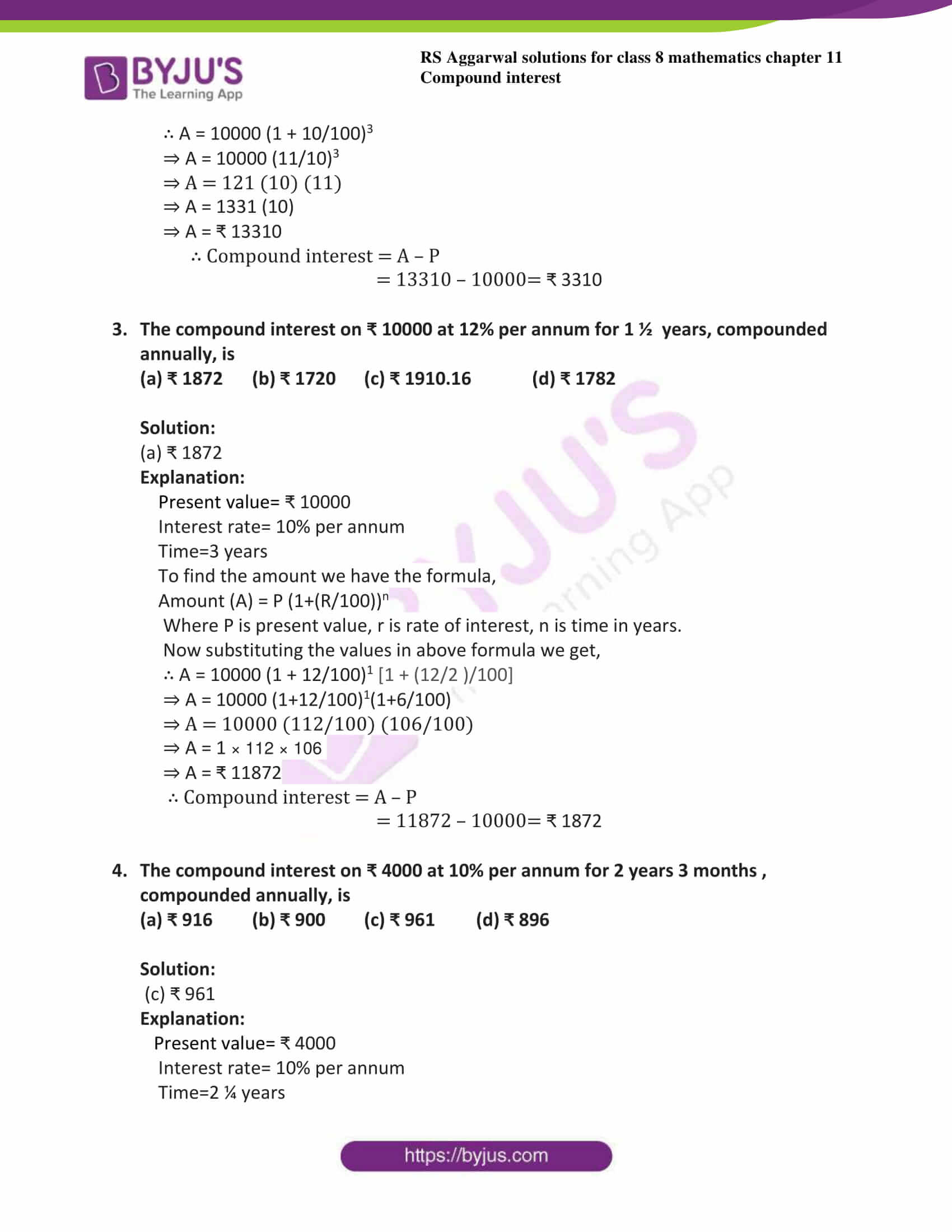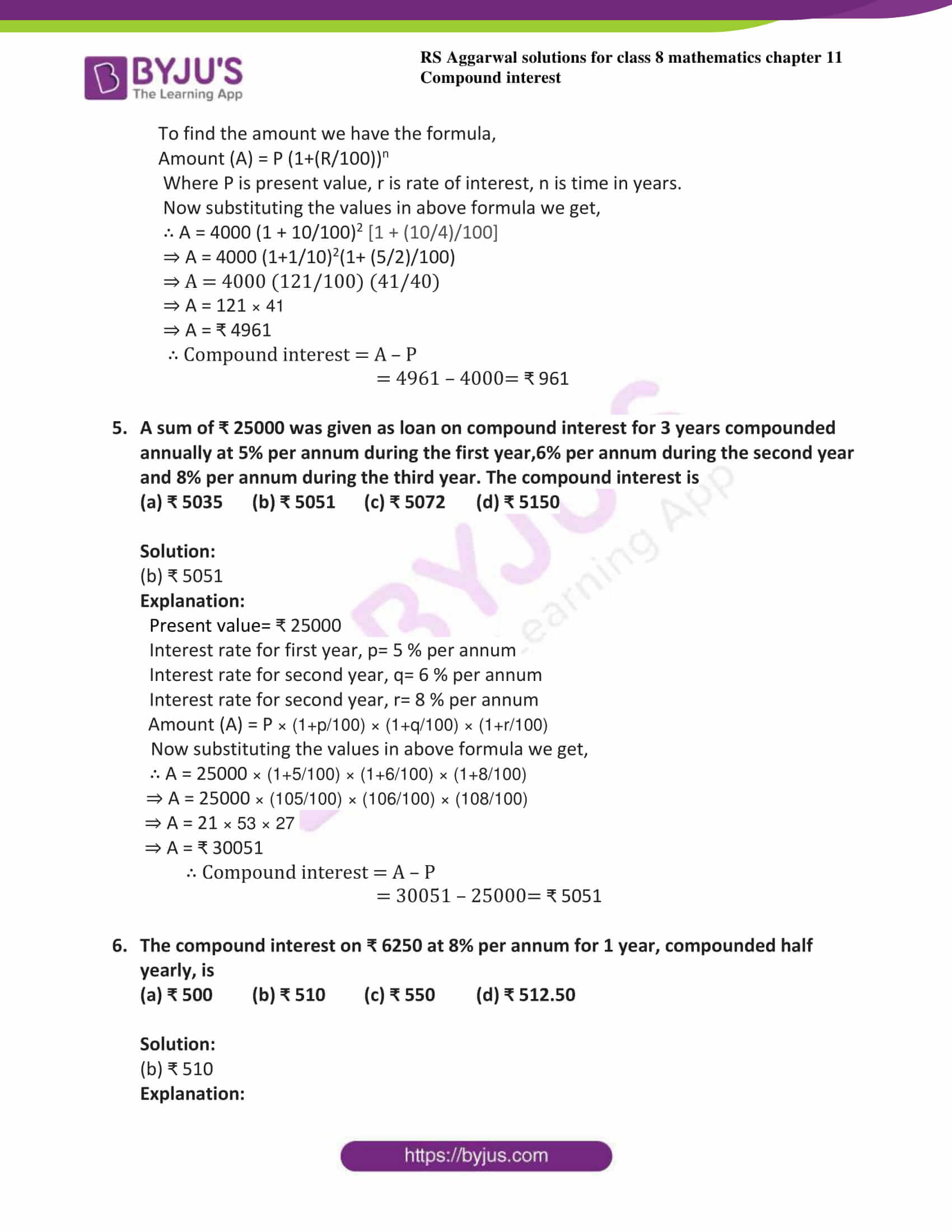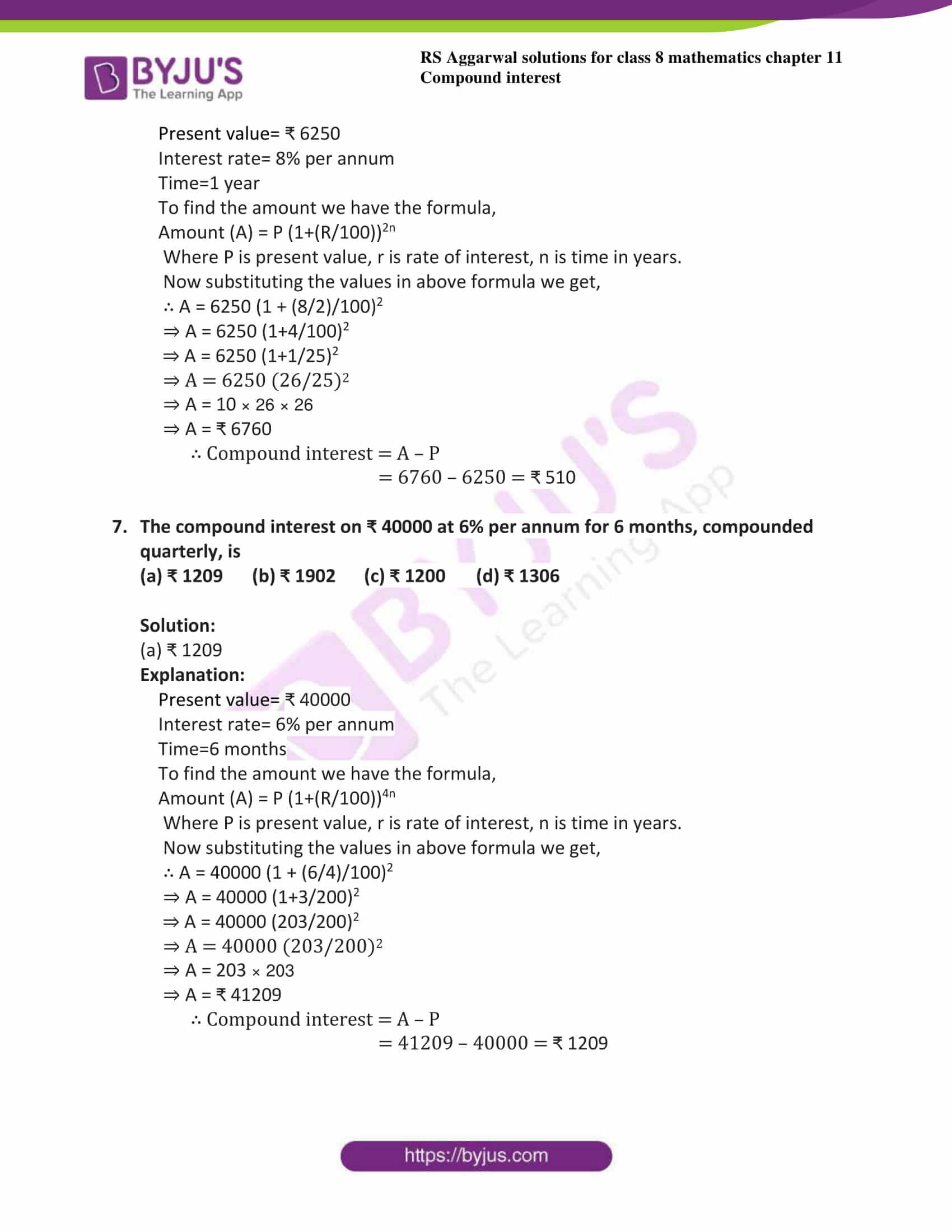# RS Aggarwal Solutions for Class 8 Chapter 11 - Compound Interest Exercise 11D

To acquire good scores, students can refer and download the RS Aggarwal Solutions for the Exercise 11D of Class 8 Maths Chapter 11, Compound Interest from the link available here. To accomplish their aim, BYJU’S experts solved these problems with the ultimate concern. This exercise bears multiple-choice questions, and this will help the students to get more knowledge about the topic. By following the RS Aggarwal Solutions for class 8, students will be able to grasp the concepts correctly.

## Download PDF of RS Aggarwal Solutions for Class 8 Chapter 11 – Compound Interest Exercise 11D### Access answers to Maths RS Aggarwal Solutions for Class 8 Chapter 11 – Compound Interest Exercise 11D

Select the correct answer in each of the following:

1. The compound interest on ₹ 5000 at 8% per annum for 2 years, compounded annually, is

(a) ₹ 800 (b) ₹ 825 (c) ₹ 832 (d) ₹ 850

Solution:

(c) ₹ 832

Explanation:

Present value= ₹ 5000

Interest rate= 8% per annum

Time=2 years

To find the amount we have the formula,

Amount (A) = P (1+(R/100))n

Where P is present value, r is rate of interest, n is time in years.

Now substituting the values in above formula we get,

∴ A = 5000 (1 + 8/100)2

⇒ A = 5000 (27/25)2

⇒ A = 200 (27 × 27/25)

⇒ A = 27 (27) (8)

⇒ A = ₹ 5832

∴ Compound interest = A – P

= 5832 – 5000= ₹ 832

2. The compound interest on ₹ 10000 at 10% per annum for 3 years, compounded annually, is

(a) ₹ 1331 (b) ₹ 3310 (c) ₹ 3130 (d) ₹ 13310

Solution:

(b) ₹ 3310

Explanation:

Present value= ₹ 10000

Interest rate= 10% per annum

Time=3 years

To find the amount we have the formula,

Amount (A) = P (1+(R/100))n

Where P is present value, r is rate of interest, n is time in years.

Now substituting the values in above formula we get,

∴ A = 10000 (1 + 10/100)3

⇒ A = 10000 (11/10)3

⇒ A = 121 (10) (11)

⇒ A = 1331 (10)

⇒ A = ₹ 13310

∴ Compound interest = A – P

= 13310 – 10000= ₹ 3310

3. The compound interest on ₹ 10000 at 12% per annum for 1 ½ years, compounded annually, is

(a) ₹ 1872 (b) ₹ 1720 (c) ₹ 1910.16 (d) ₹ 1782

Solution:

(a) ₹ 1872

Explanation:

Present value= ₹ 10000

Interest rate= 10% per annum

Time=3 years

To find the amount we have the formula,

Amount (A) = P (1+(R/100))n

Where P is present value, r is rate of interest, n is time in years.

Now substituting the values in above formula we get,

∴ A = 10000 (1 + 12/100)1 [1 + (12/2 )/100]

⇒ A = 10000 (1+12/100)1(1+6/100)

⇒ A = 10000 (112/100) (106/100)

⇒ A = 1 × 112 × 106

⇒ A = ₹ 11872

∴ Compound interest = A – P

= 11872 – 10000= ₹ 1872

4. The compound interest on ₹ 4000 at 10% per annum for 2 years 3 months , compounded annually, is

(a) ₹ 916 (b) ₹ 900 (c) ₹ 961 (d) ₹ 896

Solution:

(c) ₹ 961

Explanation:

Present value= ₹ 4000

Interest rate= 10% per annum

Time=2 ¼ years

To find the amount we have the formula,

Amount (A) = P (1+(R/100))n

Where P is present value, r is rate of interest, n is time in years.

Now substituting the values in above formula we get,

∴ A = 4000 (1 + 10/100)2 [1 + (10/4)/100]

⇒ A = 4000 (1+1/10)2(1+ (5/2)/100)

⇒ A = 4000 (121/100) (41/40)

⇒ A = 121 × 41

⇒ A = ₹ 4961

∴ Compound interest = A – P

= 4961 – 4000= ₹ 961

5. A sum of ₹ 25000 was given as loan on compound interest for 3 years compounded annually at 5% per annum during the first year,6% per annum during the second year and 8% per annum during the third year. The compound interest is

(a) ₹ 5035 (b) ₹ 5051 (c) ₹ 5072 (d) ₹ 5150

Solution:

(b) ₹ 5051

Explanation:

Present value= ₹ 25000

Interest rate for first year, p= 5 % per annum

Interest rate for second year, q= 6 % per annum

Interest rate for second year, r= 8 % per annum

Amount (A) = P × (1+p/100) × (1+q/100) × (1+r/100)

Now substituting the values in above formula we get,

∴ A = 25000 × (1+5/100) × (1+6/100) × (1+8/100)

⇒ A = 25000 × (105/100) × (106/100) × (108/100)

⇒ A = 21 × 53 × 27

⇒ A = ₹ 30051

∴ Compound interest = A – P

= 30051 – 25000= ₹ 5051

6. The compound interest on ₹ 6250 at 8% per annum for 1 year, compounded half yearly, is

(a) ₹ 500 (b) ₹ 510 (c) ₹ 550 (d) ₹ 512.50

Solution:

(b) ₹ 510

Explanation:

Present value= ₹ 6250

Interest rate= 8% per annum

Time=1 year

To find the amount we have the formula,

Amount (A) = P (1+(R/100))2n

Where P is present value, r is rate of interest, n is time in years.

Now substituting the values in above formula we get,

∴ A = 6250 (1 + (8/2)/100)2

⇒ A = 6250 (1+4/100)2

⇒ A = 6250 (1+1/25)2

⇒ A = 6250 (26/25)2

⇒ A = 10 × 26 × 26

⇒ A = ₹ 6760

∴ Compound interest = A – P

= 6760 – 6250 = ₹ 510

7. The compound interest on ₹ 40000 at 6% per annum for 6 months, compounded quarterly, is

(a) ₹ 1209 (b) ₹ 1902 (c) ₹ 1200 (d) ₹ 1306

Solution:

(a) ₹ 1209

Explanation:

Present value= ₹ 40000

Interest rate= 6% per annum

Time=6 months

To find the amount we have the formula,

Amount (A) = P (1+(R/100))4n

Where P is present value, r is rate of interest, n is time in years.

Now substituting the values in above formula we get,

∴ A = 40000 (1 + (6/4)/100)2

⇒ A = 40000 (1+3/200)2

⇒ A = 40000 (203/200)2

⇒ A = 40000 (203/200)2

⇒ A = 203 × 203

⇒ A = ₹ 41209

∴ Compound interest = A – P

= 41209 – 40000 = ₹ 1209

## RS Aggarwal Solutions for Class 8 Maths Chapter 11 – Compound Interest Exercise 11D

Exercise 11D of RS Aggarwal Solutions for Chapter 11, Compound Interest covers all the topics related to compound interest and methods of solving compound interest using the formulae. Some of the topics focused prior to exercise 11D include the following.

• Definition of compound interest
• To find compound interest when interest is compounded annually
• To find compound interest when interest is compounded half-yearly
• Calculating the compound interest by using formulae
• Applications of compound interest formula

The RS Aggarwal Solutions can help the students in practising and learning each and every concept as it provides solutions to all questions asked in RS Aggarwal textbook.